Platform: All Platforms Applies to: , Acoustics Module, COMSOL Multiphysics®, MEMS Module, Plasma Module, RF Module, Semiconductor Module, Structural Mechanics Module, Wave Optics Module Versions: All versions

## Problem Description

How does COMSOL Multiphysics handle complex-valued numbers and problems in the frequency domain?

Should I use the RMS, the peak, or the instantaneous value to specify sources in models being solved in the frequency domain, such as electromagnetic or acoustic models?

How can I specify the phase angle?

## Solution

The COMSOL Multiphysics formulation uses complex fields when solving time-harmonic problems in electromagnetics, acoustics, and structural mechanics, or any other physics where the Frequency Domain study type is being used. For example, when solving electromagnetic problems, one is often solving for the electric field: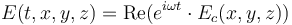where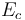is the complex electric field that you are entering,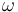is the angular frequency,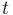is time, and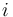is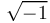.

Whenever you specify a source field you can input a complex number (a phasor) such as `Ec` where `abs(Ec)` is the peak value, and `real(Ec)` gives you the instantaneous value at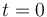.

This applies for any time-harmonic input. So, to define for instance an external current density, a magnetic field, an acoustic pressure, or an electric potential, with an RMS value of 1 and a phase angle of 30°, enter the expression:

`sqrt(2)*exp(i*30[deg])`

or, alternatively:

`sqrt(2)*(cos(30[deg])+i*sin(30[deg]))`

The following functions are useful when dealing with complex-valued numbers:

`real()` Returns the real component of the argument.

`imag()` Returns the imaginary component of the argument.

`abs()` Returns the magnitude of the complex-valued argument.

`arg()` Returns the phase.

`conj()` Returns the complex conjugate.